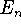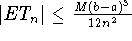Next: Exercises Up: Background Previous: Trapezoidal rule and Simpson's

### Error Bounds

The accuracy delivered by these methods is seen in the following error bounds. (Recall that the error bound is a bound on the absolute value of the difference between the actual area under the curve and its estimated value.)

For n intervals we express the error between the actual and approximate area by. From page 306 of the text we have:

Trapezoidal error:, where M is the maximum of |f''(x)| on [a,b]

Simpson's error:, where K is the maximum of |f''''(x)| on [a,b]

Sean O Anderson
Mon Nov 11 16:16:00 EST 1996